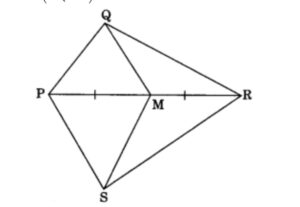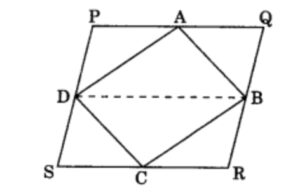## MCQ Questions of Class 9 Maths Chapter 9 Areas of Parallelogram and Triangles with Answers

Free PDF Download of CBSE Class 9 Maths Chapter 9 Areas of Parallelogram and Triangles Multiple Choice Questions with Answers.Based on Latest Exam Pattern. Students can solve NCERT Class 9 Maths Chapter 9 Areas of Parallelogram and Triangles Multiple Choice Questions with Answers to know their preparation level.

# Areas of Parallelogram and Triangles Class 9 MCQs Questions with Answers

MCQ Questions for Class 9 Maths Chapter 9 Areas of Parallelogram and Triangles with Answers

1. ABCD is a trapezium in which AB || DC. If ar(ΔABD) = 24 cm² and AB = 8 cm, then height of ΔABC is2. If Diagonals AC and BD of a quadrilateral ABCD intersect at O in such a way that ar(△AOD) = ar(△BOC). Then ABCD is a:

3. If ABCD is a parallelogram, AE ⊥ DC and CF ⊥ AD. If AB = 10 cm, AE = 6 cm and CF = 5 cm, then AD is equal to:

4. PQRS is a parallelogram. If X and Y are the mid-points of PQ and SR and diagonal SQ is joined, then ar(XQRY) : ar(ΔQSR) is5. If a triangle and a parallelogram are on the same base and between same parallels, then the ratio of the area of the triangle to the area of the parallelogram will be:

6. In quadrilateral PQRS, M is the mid-point of PR. If ar(SMQR) = 18 cm², then ar(PQMS) is7. In a triangle ABC, E is the mid-point of median AD. Then

8. A median of a triangle divides it into two

9. A, B, C and D are the mid-points of sides of parallelogram PQRS. If ar(PQRS) = 36 cm², then ar(ABCD) is10. If E, F, G and H are the mid-points of the sides of a parallelogram ABCD, respectively, then ar (EFGH) is equal to:

11. ABCD is a quadrilateral whose diagonal AC divides it in two parts of equal area, then ABCD is a

12. The area of the figure formed by joining the mid-points of the adjacent sides of a rhombus with diagonals 12 cm and 16 cm is

13. D and E are the mid-points of BC and AD respectively. If ar(ΔABC) = 12 cm², then ar(ΔBDE) is

14. If ABCD and EFGH are two parallelograms between same parallel lines and on the same base, then:

15. If Diagonals AC and BD of a trapezium ABCD with AB || DC intersect each other at O. Then,

16. If Diagonals AC and BD of a trapezium ABCD with AB || DC intersect each other at O. Then,

17. If P and Q are any two points lying on the sides DC and AD respectively of a parallelogram ABCD, then:

18. If D and E are points on sides AB and AC respectively of ΔABC such that ar(DBC) = ar(EBC). Then:

19. If P and Q are any two points lying on the sides DC and AD respectively of a parallelogram ABCD, then:

20. The median of a triangle divides it into two

Hope the information shed above regarding MCQ Questions for Class 9 Maths Chapter 9 Areas of Parallelogram and Triangles with Answers Pdf free download has been useful to an extent. If you have any other queries of CBSE Class 9 Maths Chapter 9 Areas of Parallelogram and Triangles MCQs Multiple Choice Questions with Answers, feel free to comment below so that we can revert back to us at the earliest possible

By Team Study Rate